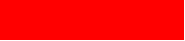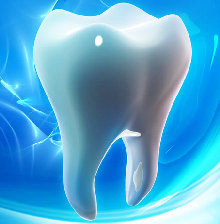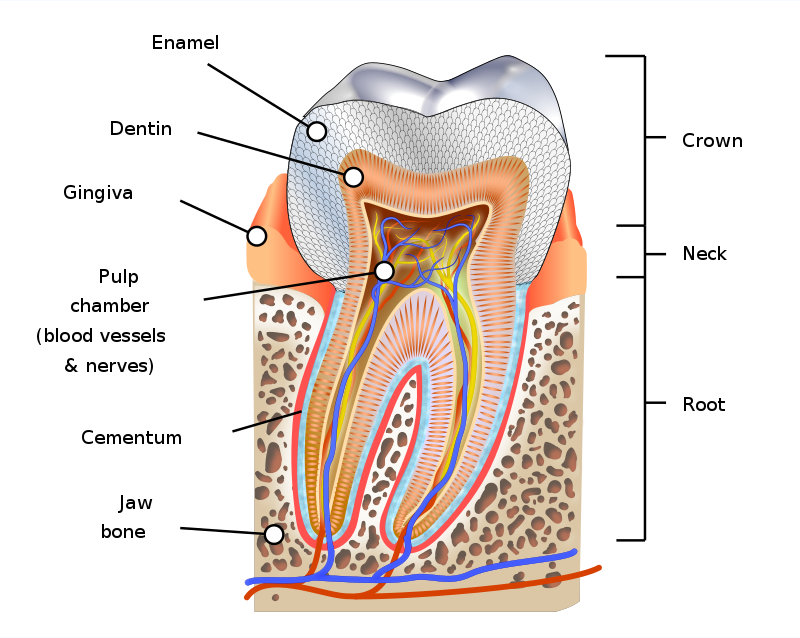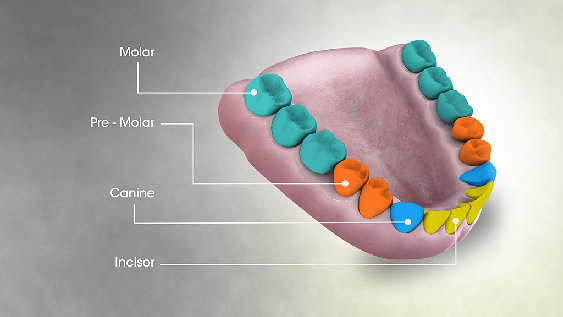SUPERDENTThe maxillary teeth are the maxillary central incisors (teeth 8 and 9 in the diagram), maxillary lateral incisors (7 and 10), maxillary canines (6 and 11), maxillary first premolars (5 and 12), maxillary second premolars (4 and 13), maxillary first molars (3 and 14), maxillary second molars (2 and 15), and maxillary third molars (1 and 16). The mandibular teeth are the mandibular central incisors (24 and 25), mandibular lateral incisors (23 and 26), mandibular canines (22 and 27), mandibular first premolars (21 and 28), mandibular second premolars (20 and 29), mandibular first molars (19 and 30), mandibular second molars (18 and 31), and mandibular third molars (17 and 32). Third molars are commonly called "wisdom teeth" and may never erupt into the mouth or form at all.DENTCAP